SNAP Library 6.0, Developer Reference  2020-12-09 16:24:20 SNAP, a general purpose, high performance system for analysis and manipulation of large networks
TGraphKey Class Reference

Small Directed Graphs. More...

`#include <ghash.h>`

Collaboration diagram for TGraphKey: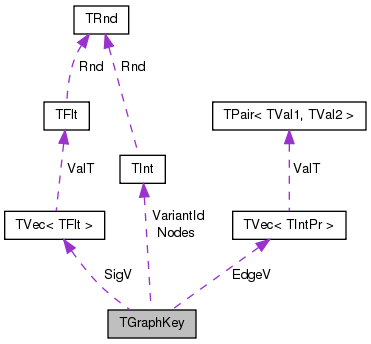[legend]

## Public Member Functions

TGraphKey ()

TGraphKey (const TSFltV &GraphSigV)

TGraphKey (const TIntV &GraphSigV)

TGraphKey (const TFltV &GraphSigV)

TGraphKey (const TGraphKey &GraphKey)

TGraphKey (TSIn &SIn)

void Save (TSOut &SOut) const

TGraphKeyoperator= (const TGraphKey &GraphKey)

bool operator== (const TGraphKey &GraphKey) const

int GetPrimHashCd () const

int GetSecHashCd () const

int GetNodes () const
Returns the number of nodes in the graph. More...

int GetEdges () const
Returns the number of edges in the graph. More...

int GetSigLen () const
Returns the length of the signature vector of a graph. More...

int GetVariant () const
Returns the graph variant Id. More...

void SetVariant (const int &Variant)
Sets the Variant Id of a given graph. More...

void SetEdgeV (const TIntPrV &EdgeIdV)
Returns a vector of directed edges of a graph. More...

PNGraph GetNGraph () const
Returns the directed graph stored in the GraphKey object. More...

void TakeGraph (const PNGraph &Graph)
Creates a key from a given directed graph. More...

void TakeGraph (const PNGraph &Graph, TIntPrV &NodeMap)
Creates a key from a given directed graph. More...

void TakeSig (const PNGraph &Graph, const int &MnSvdGraph, const int &MxSvdGraph)
Creates a signature for a given directed graph. More...

void SaveTxt (FILE *F) const
Saves the graph as a list of edges. More...

void SaveGViz (const TStr &OutFNm, const TStr &Desc=TStr(), const TStr &NodeAttrs="", const int &Size=-1) const
Saves the graph to the .DOT file format used by GraphViz. More...

void DrawGViz (const TStr &OutFNm, const TStr &Desc=TStr(), const TStr &NodeAttrs="", const int &Size=-1) const
Saves the graph to the .DOT file format and calls GraphViz to draw it. More...

## Static Public Member Functions

static bool IsIsomorph (const TGraphKey &Key1, const TGraphKey &Key2, const TIntV &NodeIdMap)
Checks whether directed graph Key1 is isomorphic to the directed graph Key2 under node Id permutation NodeIdMap. More...

static bool IsIsomorph (const TGraphKey &Key1, const TGraphKey &Key2, const TVec< TIntV > &NodeIdMapV)
Checks whether directed graph Key1 is isomorphic to the directed graph Key2 under all the permutations of node Ids stored in NodeIdMapV. More...

static bool IsIsomorph (const TGraphKey &Key1, const TGraphKey &Key2, const TVec< TIntV > &NodeIdMapV, int &IsoPermId)
Checks whether directed graph Key1 is isomorphic to the directed graph Key2 under all the permutations of node Ids stored in NodeIdMapV and returns the Id of the permutation of node Ids (IsoPermId) which makes the two graphs isomorphic. More...

TInt Nodes

TIntPrV EdgeV

TFltV SigV

TInt VariantId

## Static Public Attributes

static const int RoundTo = 4

## Detailed Description

Small Directed Graphs.

These graphs are used as keys of the TGHash graph hash table. The main functionality of TGraphKey is that it performs fast graph isomorphism checking to determine whether two graphs (two keys) are the same (i.e., isomorphic).

Definition at line 7 of file ghash.h.

## Constructor & Destructor Documentation

 TGraphKey::TGraphKey ( )
inline

Definition at line 17 of file ghash.h.

17 : Nodes(-1), EdgeV(), SigV(), VariantId(0) { }
TFltV SigV
Definition: ghash.h:14
TInt Nodes
Definition: ghash.h:12
TIntPrV EdgeV
Definition: ghash.h:13
TInt VariantId
Definition: ghash.h:15
 TGraphKey::TGraphKey ( const TSFltV & GraphSigV )

Definition at line 5 of file ghash.cpp.

5  : Nodes(-1), EdgeV(), SigV(), VariantId(0) {
6  SigV.Gen(GraphSigV.Len());
7  for (int i = 0; i < GraphSigV.Len(); i++) {
8  SigV[i] = TFlt(TMath::Round(GraphSigV[i], RoundTo));
9  }
10 }
TFltV SigV
Definition: ghash.h:14
TSizeTy Len() const
Returns the number of elements in the vector.
Definition: ds.h:575
TInt Nodes
Definition: ghash.h:12
Definition: dt.h:1386
static const int RoundTo
Definition: ghash.h:9
static double Round(const double &Val)
Definition: xmath.h:16
TIntPrV EdgeV
Definition: ghash.h:13
TInt VariantId
Definition: ghash.h:15
void Gen(const TSizeTy &_Vals)
Constructs a vector (an array) of _Vals elements.
Definition: ds.h:523

Here is the call graph for this function: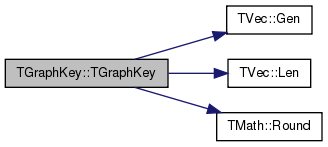TGraphKey::TGraphKey ( const TIntV & GraphSigV )

Definition at line 12 of file ghash.cpp.

References TVec< TVal, TSizeTy >::Gen(), TVec< TVal, TSizeTy >::Len(), and SigV.

12  : Nodes(-1), EdgeV(), SigV(), VariantId(0) {
13  SigV.Gen(GraphSigV.Len());
14  for (int i = 0; i < GraphSigV.Len(); i++) {
15  SigV[i] = TFlt(GraphSigV[i]());
16  }
17 }
TFltV SigV
Definition: ghash.h:14
TSizeTy Len() const
Returns the number of elements in the vector.
Definition: ds.h:575
TInt Nodes
Definition: ghash.h:12
Definition: dt.h:1386
TIntPrV EdgeV
Definition: ghash.h:13
TInt VariantId
Definition: ghash.h:15
void Gen(const TSizeTy &_Vals)
Constructs a vector (an array) of _Vals elements.
Definition: ds.h:523

Here is the call graph for this function:TGraphKey::TGraphKey ( const TFltV & GraphSigV )

Definition at line 19 of file ghash.cpp.

19  : Nodes(-1), EdgeV(), SigV(), VariantId(0) {
20  SigV.Gen(GraphSigV.Len());
21  for (int i = 0; i < GraphSigV.Len(); i++) {
22  SigV[i] = TFlt(TMath::Round(GraphSigV[i], RoundTo));
23  }
24 }
TFltV SigV
Definition: ghash.h:14
TSizeTy Len() const
Returns the number of elements in the vector.
Definition: ds.h:575
TInt Nodes
Definition: ghash.h:12
Definition: dt.h:1386
static const int RoundTo
Definition: ghash.h:9
static double Round(const double &Val)
Definition: xmath.h:16
TIntPrV EdgeV
Definition: ghash.h:13
TInt VariantId
Definition: ghash.h:15
void Gen(const TSizeTy &_Vals)
Constructs a vector (an array) of _Vals elements.
Definition: ds.h:523

Here is the call graph for this function:TGraphKey::TGraphKey ( const TGraphKey & GraphKey )

Definition at line 26 of file ghash.cpp.

26  : Nodes(GraphKey.Nodes),
27  EdgeV(GraphKey.EdgeV), SigV(GraphKey.SigV), VariantId(GraphKey.VariantId) {
28 }
TFltV SigV
Definition: ghash.h:14
TInt Nodes
Definition: ghash.h:12
TIntPrV EdgeV
Definition: ghash.h:13
TInt VariantId
Definition: ghash.h:15
 TGraphKey::TGraphKey ( TSIn & SIn )

Definition at line 30 of file ghash.cpp.

30 : Nodes(SIn), EdgeV(SIn), SigV(SIn), VariantId(SIn) { }
TFltV SigV
Definition: ghash.h:14
TInt Nodes
Definition: ghash.h:12
TIntPrV EdgeV
Definition: ghash.h:13
TInt VariantId
Definition: ghash.h:15

## Member Function Documentation

 void TGraphKey::DrawGViz ( const TStr & OutFNm, const TStr & Desc = `TStr()`, const TStr & NodeAttrs = `""`, const int & Size = `-1` ) const

Saves the graph to the .DOT file format and calls GraphViz to draw it.

Output type is determined by the OutFNm file extension (.ps, .png).

Definition at line 180 of file ghash.cpp.

References TStr::GetFMid(), TSnap::TSnapDetail::GVizDoLayout(), gvlDot, and SaveGViz().

180  {
181  const TStr DotFNm = OutFNm.GetFMid()+".dot";
182  SaveGViz(DotFNm, Desc, NodeAttrs, Size);
183  TSnap::TSnapDetail::GVizDoLayout(DotFNm, OutFNm, gvlDot);
184 }
TStr GetFMid() const
Definition: dt.cpp:1403
void GVizDoLayout(const TStr &GraphInFNm, TStr OutFNm, const TGVizLayout &Layout)
Runs GraphViz layout engine over a graph saved in the file GraphInFNm with output saved to OutFNm...
Definition: gviz.cpp:5
Definition: gviz.h:3
void SaveGViz(const TStr &OutFNm, const TStr &Desc=TStr(), const TStr &NodeAttrs="", const int &Size=-1) const
Saves the graph to the .DOT file format used by GraphViz.
Definition: ghash.cpp:154
Definition: dt.h:412

Here is the call graph for this function: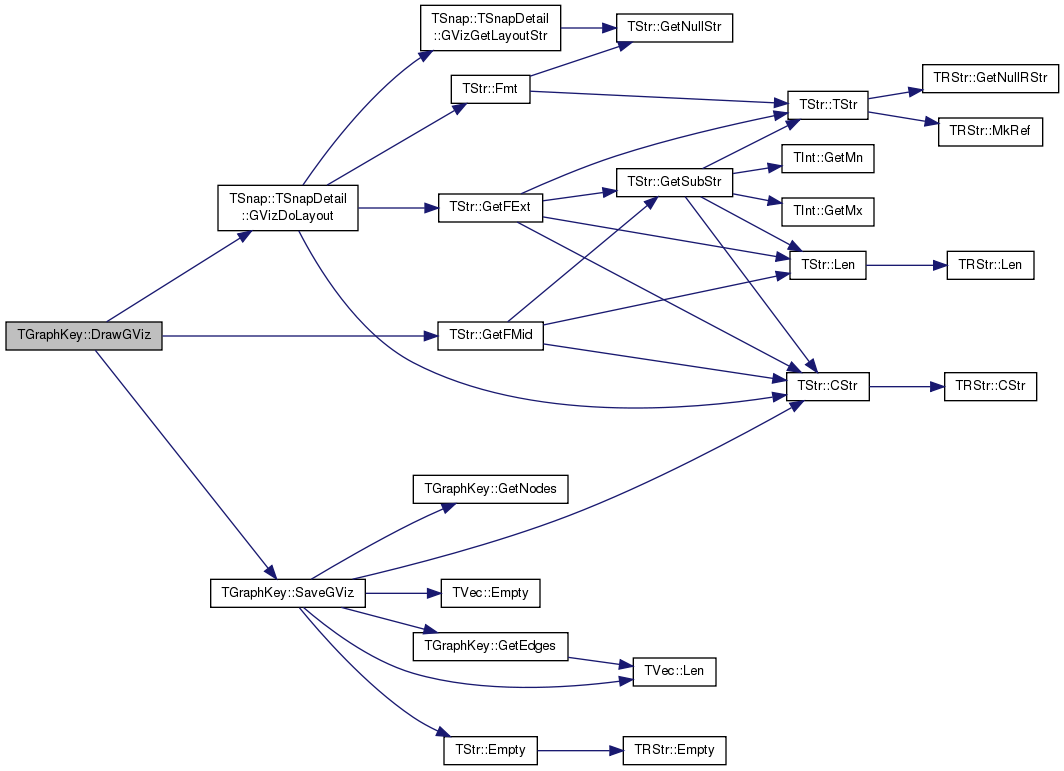int TGraphKey::GetEdges ( ) const
inline

Returns the number of edges in the graph.

Definition at line 33 of file ghash.h.

References TVec< TVal, TSizeTy >::Len().

Referenced by TGHash< TDat >::DrawGViz(), GetNGraph(), SaveGViz(), SaveTxt(), and TGHash< TDat >::SaveTxt().

33 { return EdgeV.Len(); }
TSizeTy Len() const
Returns the number of elements in the vector.
Definition: ds.h:575
TIntPrV EdgeV
Definition: ghash.h:13

Here is the call graph for this function: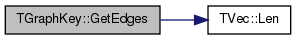Here is the caller graph for this function: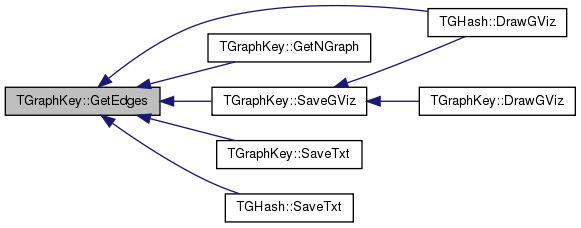PNGraph TGraphKey::GetNGraph ( ) const

Returns the directed graph stored in the GraphKey object.

Definition at line 47 of file ghash.cpp.

47  {
48  PNGraph G = TNGraph::New();
49  for (int i = 0; i < GetNodes(); i++) G->AddNode(i);
50  for (int e = 0; e < GetEdges(); e++) {
52  }
53  G->Defrag();
54  return G;
55 }
int GetEdges() const
Returns the number of edges in the graph.
Definition: ghash.h:33
static PNGraph New()
Static constructor that returns a pointer to the graph. Call: PNGraph Graph = TNGraph::New().
Definition: graph.h:481
TIntPrV EdgeV
Definition: ghash.h:13
int GetNodes() const
Returns the number of nodes in the graph.
Definition: ghash.h:31
Definition: bd.h:196

Here is the call graph for this function: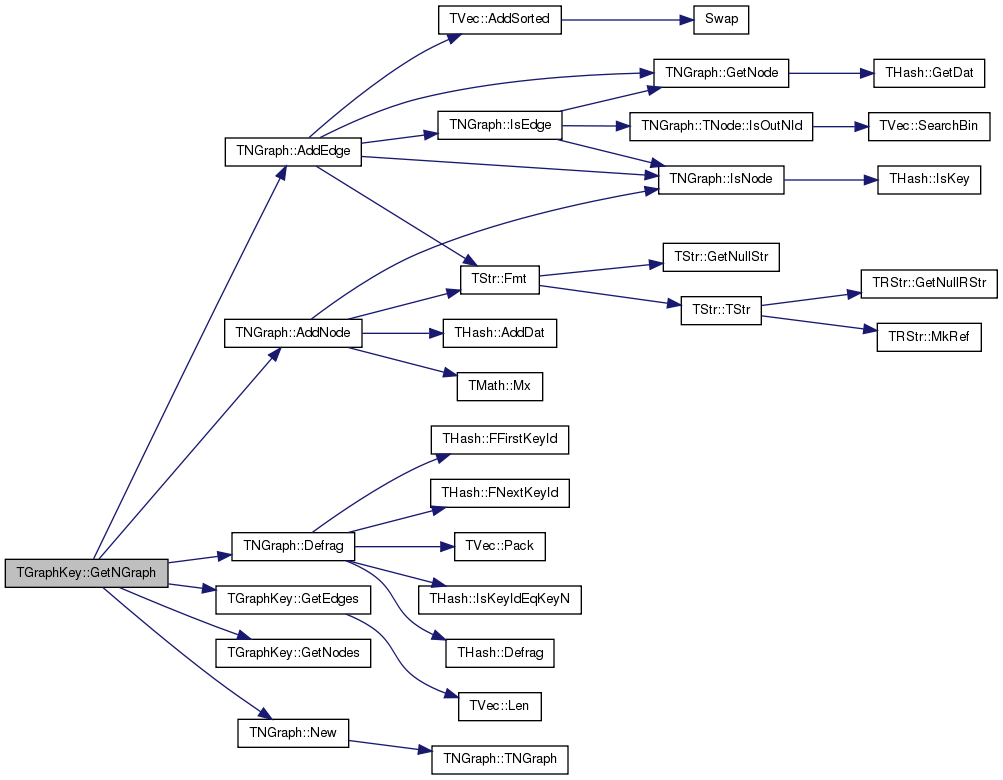int TGraphKey::GetNodes ( ) const
inline

Returns the number of nodes in the graph.

Definition at line 31 of file ghash.h.

References Nodes.

31 { return Nodes; }
TInt Nodes
Definition: ghash.h:12

Here is the caller graph for this function: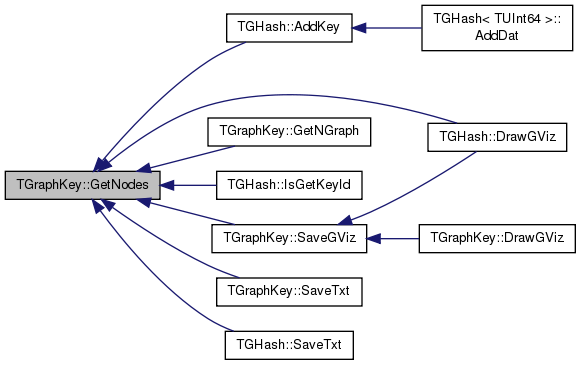int TGraphKey::GetPrimHashCd ( ) const
inline

Definition at line 27 of file ghash.h.

References TVec< TVal, TSizeTy >::GetPrimHashCd(), and VariantId.

27 { return abs(SigV.GetPrimHashCd() ^ VariantId); }
int GetPrimHashCd() const
Returns primary hash code of the vector. Used by THash.
Definition: ds.h:999
TFltV SigV
Definition: ghash.h:14
TInt VariantId
Definition: ghash.h:15

Here is the call graph for this function: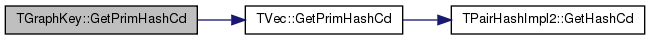int TGraphKey::GetSecHashCd ( ) const
inline

Definition at line 28 of file ghash.h.

References TVec< TVal, TSizeTy >::GetSecHashCd().

28 { return abs(SigV.GetSecHashCd() ^ VariantId<<8); }
TFltV SigV
Definition: ghash.h:14
int GetSecHashCd() const
Returns secondary hash code of the vector. Used by THash.
Definition: ds.h:1011
TInt VariantId
Definition: ghash.h:15

Here is the call graph for this function: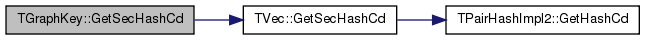int TGraphKey::GetSigLen ( ) const
inline

Returns the length of the signature vector of a graph.

Signature is a set of statistics that is used to quickly determine whether the two graphs could be isomorphic. Graphs that differ in their signatures are guaranteed to be non-isomorphic while graphs with identical signatures could still be isomorphic.

Definition at line 40 of file ghash.h.

References TVec< TVal, TSizeTy >::Len().

40 { return SigV.Len(); }
TFltV SigV
Definition: ghash.h:14
TSizeTy Len() const
Returns the number of elements in the vector.
Definition: ds.h:575

Here is the call graph for this function: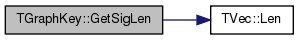int TGraphKey::GetVariant ( ) const
inline

Returns the graph variant Id.

When the hash table contains multiple non-isomorphic graphs with identical signatures we assign each a unique VariantId.

Definition at line 45 of file ghash.h.

References VariantId.

45 { return VariantId; }
TInt VariantId
Definition: ghash.h:15
 bool TGraphKey::IsIsomorph ( const TGraphKey & Key1, const TGraphKey & Key2, const TIntV & NodeIdMap )
static

Checks whether directed graph Key1 is isomorphic to the directed graph Key2 under node Id permutation NodeIdMap.

The function does not consider all possible permutations (mappings) between node Ids but only considers mapping in NodeIdMap.

Definition at line 186 of file ghash.cpp.

References EdgeV, TVec< TVal, TSizeTy >::Len(), Nodes, and TVec< TVal, TSizeTy >::SearchBin().

186  {
187  const TIntPrV& EdgeV1 = Key1.EdgeV;
188  const TIntPrV& EdgeV2 = Key2.EdgeV;
189  if (Key1.Nodes != Key2.Nodes || EdgeV1.Len() != EdgeV2.Len()) { return false; }
190  for (int e1 = 0; e1 < EdgeV1.Len(); e1++) {
191  const TIntPr Edge2(NodeIdMap[EdgeV1[e1].Val1], NodeIdMap[EdgeV1[e1].Val2]);
192  if (EdgeV2.SearchBin(Edge2) == -1) return false;
193  }
194  return true;
195 }
TSizeTy Len() const
Returns the number of elements in the vector.
Definition: ds.h:575
TInt Nodes
Definition: ghash.h:12
TIntPrV EdgeV
Definition: ghash.h:13
TSizeTy SearchBin(const TVal &Val) const
Returns the position of an element with value Val.
Definition: ds.h:1519
Definition: ds.h:32

Here is the call graph for this function:Here is the caller graph for this function: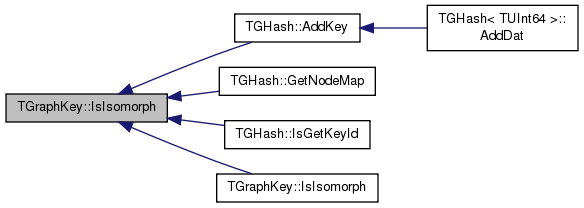bool TGraphKey::IsIsomorph ( const TGraphKey & Key1, const TGraphKey & Key2, const TVec< TIntV > & NodeIdMapV )
static

Checks whether directed graph Key1 is isomorphic to the directed graph Key2 under all the permutations of node Ids stored in NodeIdMapV.

Definition at line 197 of file ghash.cpp.

References IsIsomorph().

197  {
198  int IsoPermId;
199  return IsIsomorph(Key1, Key2, NodeIdMapV, IsoPermId);
200 }
static bool IsIsomorph(const TGraphKey &Key1, const TGraphKey &Key2, const TIntV &NodeIdMap)
Checks whether directed graph Key1 is isomorphic to the directed graph Key2 under node Id permutation...
Definition: ghash.cpp:186

Here is the call graph for this function:bool TGraphKey::IsIsomorph ( const TGraphKey & Key1, const TGraphKey & Key2, const TVec< TIntV > & NodeIdMapV, int & IsoPermId )
static

Checks whether directed graph Key1 is isomorphic to the directed graph Key2 under all the permutations of node Ids stored in NodeIdMapV and returns the Id of the permutation of node Ids (IsoPermId) which makes the two graphs isomorphic.

Definition at line 202 of file ghash.cpp.

References EdgeV, TVec< TVal, TSizeTy >::Len(), and Nodes.

202  {
203  const TIntPrV& EdgeV1 = Key1.EdgeV;
204  const TIntPrV& EdgeV2 = Key2.EdgeV;
205  //for (int i = 0; i < EdgeV1.Len(); i++) printf("\t%d - %d\n", EdgeV1[i].Val1, EdgeV1[i].Val2); printf("\n");
206  //for (int i = 0; i < EdgeV2.Len(); i++) printf("\t%d - %d\n", EdgeV2[i].Val1, EdgeV2[i].Val2);
207  if (Key1.Nodes != Key2.Nodes || EdgeV1.Len() != EdgeV2.Len()) return false;
208  const int Nodes = NodeIdMapV.Len();
211  for (int i = 0; i < EdgeV2.Len(); i++) {
212  AdjMtx2[EdgeV2[i].Val1*Nodes + EdgeV2[i].Val2] = 1;
213  }
214  for (int perm = 0; perm < NodeIdMapV.Len(); perm++) {
215  const TIntV& NodeIdMap = NodeIdMapV[perm];
216  bool IsIso = true;
217  for (int e1 = 0; e1 < EdgeV1.Len(); e1++) {
218  const int NId1 = NodeIdMap[EdgeV1[e1].Val1];
219  const int NId2 = NodeIdMap[EdgeV1[e1].Val2];
220  if (AdjMtx2[NId1*Nodes + NId2] != 1) {
221  IsIso = false; break; }
222  }
223  if (IsIso) {
224  IsoPermId = perm;
225  return true; }
226  }
227  IsoPermId = -1;
228  return false;
229 }
TSizeTy Len() const
Returns the number of elements in the vector.
Definition: ds.h:575
TInt Nodes
Definition: ghash.h:12
TIntPrV EdgeV
Definition: ghash.h:13

Here is the call graph for this function: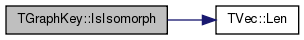TGraphKey & TGraphKey::operator= ( const TGraphKey & GraphKey )

Definition at line 37 of file ghash.cpp.

References EdgeV, Nodes, SigV, and VariantId.

37  {
38  if (this != &GraphKey) {
39  Nodes = GraphKey.Nodes;
40  EdgeV = GraphKey.EdgeV;
41  SigV = GraphKey.SigV;
42  VariantId = GraphKey.VariantId;
43  }
44  return *this;
45 }
TFltV SigV
Definition: ghash.h:14
TInt Nodes
Definition: ghash.h:12
TIntPrV EdgeV
Definition: ghash.h:13
TInt VariantId
Definition: ghash.h:15
 bool TGraphKey::operator== ( const TGraphKey & GraphKey ) const
inline

Definition at line 25 of file ghash.h.

References SigV, and VariantId.

25 { return SigV==GraphKey.SigV && VariantId==GraphKey.VariantId; }
TFltV SigV
Definition: ghash.h:14
TInt VariantId
Definition: ghash.h:15
 void TGraphKey::Save ( TSOut & SOut ) const

Definition at line 32 of file ghash.cpp.

References EdgeV, Nodes, TVec< TVal, TSizeTy >::Save(), TInt::Save(), SigV, and VariantId.

32  {
33  Nodes.Save(SOut); EdgeV.Save(SOut);
34  SigV.Save(SOut); VariantId.Save(SOut);
35 }
TFltV SigV
Definition: ghash.h:14
void Save(TSOut &SOut) const
Definition: dt.h:1153
TInt Nodes
Definition: ghash.h:12
void Save(TSOut &SOut) const
Definition: ds.h:954
TIntPrV EdgeV
Definition: ghash.h:13
TInt VariantId
Definition: ghash.h:15

Here is the call graph for this function:void TGraphKey::SaveGViz ( const TStr & OutFNm, const TStr & Desc = `TStr()`, const TStr & NodeAttrs = `""`, const int & Size = `-1` ) const

Saves the graph to the .DOT file format used by GraphViz.

Use ".dot" as file extension for OutFNm.

Definition at line 154 of file ghash.cpp.

Referenced by DrawGViz(), and TGHash< TDat >::DrawGViz().

154  {
155  FILE *F = fopen(OutFNm.CStr(), "wt");
156  fprintf(F, "/*****\n");
157  fprintf(F, " Graph (%d, %d)\n", GetNodes(), GetEdges());
158  //if (! Desc.Empty()) fprintf(F, " %s\n", Desc.CStr());
159  fprintf(F, "*****/\n\n");
160  fprintf(F, "digraph G {\n");
161  if (Size != -1) fprintf(F, " size=\"%d,%d\";\n", Size, Size);
162  fprintf(F, " graph [splines=true overlap=false]\n"); //size=\"12,10\" ratio=fill
163  if (NodeAttrs.Empty()) fprintf(F, " node [shape=ellipse, width=0.3, height=0.3]\n");
164  else fprintf(F, " node [shape=ellipse, %s]\n", NodeAttrs.CStr());
165  if (! EdgeV.Empty()) {
166  for (int e = 0; e < EdgeV.Len(); e++) {
167  fprintf(F, " %d -> %d;\n", EdgeV[e].Val1(), EdgeV[e].Val2()); }
168  } else {
169  for (int n = 0; n < Nodes; n++) { fprintf(F, " %d;\n", n); }
170  }
171  if (! Desc.Empty()) {
172  fprintf(F, " label = \"\\n%s\\n\";", Desc.CStr());
173  fprintf(F, " fontsize=24;\n");
174  }
175  fprintf(F, "}\n");
176  fclose(F);
177 }
int GetEdges() const
Returns the number of edges in the graph.
Definition: ghash.h:33
TSizeTy Len() const
Returns the number of elements in the vector.
Definition: ds.h:575
TInt Nodes
Definition: ghash.h:12
bool Empty() const
Tests whether the vector is empty.
Definition: ds.h:570
TIntPrV EdgeV
Definition: ghash.h:13
int GetNodes() const
Returns the number of nodes in the graph.
Definition: ghash.h:31
bool Empty() const
Definition: dt.h:491
char * CStr()
Definition: dt.h:479

Here is the call graph for this function: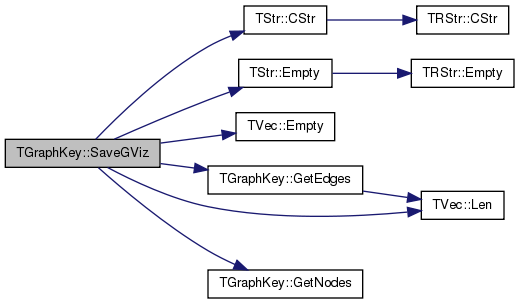Here is the caller graph for this function:void TGraphKey::SaveTxt ( FILE * F ) const

Saves the graph as a list of edges.

Definition at line 147 of file ghash.cpp.

References EdgeV, GetEdges(), GetNodes(), and TVec< TVal, TSizeTy >::Len().

147  {
148  fprintf(F, "#GraphKey. Nodes: %d. Edges: %d\n", GetNodes(), GetEdges());
149  for (int i = 0; i < EdgeV.Len(); i++) {
150  fprintf(F," %d\t%d\n", EdgeV[i].Val1(), EdgeV[i].Val2());
151  }
152 }
int GetEdges() const
Returns the number of edges in the graph.
Definition: ghash.h:33
TSizeTy Len() const
Returns the number of elements in the vector.
Definition: ds.h:575
TIntPrV EdgeV
Definition: ghash.h:13
int GetNodes() const
Returns the number of nodes in the graph.
Definition: ghash.h:31

Here is the call graph for this function:void TGraphKey::SetEdgeV ( const TIntPrV & EdgeIdV )
inline

Returns a vector of directed edges of a graph.

Definition at line 49 of file ghash.h.

49 { EdgeV = EdgeIdV; }
TIntPrV EdgeV
Definition: ghash.h:13
 void TGraphKey::SetVariant ( const int & Variant )
inline

Sets the Variant Id of a given graph.

Definition at line 47 of file ghash.h.

Referenced by TGHash< TDat >::AddKey(), TGHash< TDat >::GetNodeMap(), and TGHash< TDat >::IsGetKeyId().

47 { VariantId = Variant; }
TInt VariantId
Definition: ghash.h:15

Here is the caller graph for this function:void TGraphKey::TakeGraph ( const PNGraph & Graph )

Creates a key from a given directed graph.

Nodes get renumbered to have Ids 0...N-1. Does not create a graph signature.

Definition at line 58 of file ghash.cpp.

Referenced by TGHash< TDat >::AddKey(), TGHash< TDat >::GetNodeMap(), and TGHash< TDat >::IsGetKeyId().

58  {
59  TIntH NodeIdH;
60  for (TNGraph::TNodeI NI = Graph->BegNI(); NI < Graph->EndNI(); NI++) {
62  Nodes = Graph->GetNodes();
63  EdgeV.Gen(Nodes, 0);
64  for (TNGraph::TNodeI NI = Graph->BegNI(); NI < Graph->EndNI(); NI++) {
65  const int NewNId = NodeIdH.GetKeyId(NI.GetId());
66  for (int i = 0; i < NI.GetOutDeg(); i++) {
68  }
69  }
70  EdgeV.Sort(true);
71  EdgeV.Pack();
72 }
TPair< TInt, TInt > TIntPr
Definition: ds.h:83
TInt Nodes
Definition: ghash.h:12
void Sort(const bool &Asc=true)
Sorts the elements of the vector.
Definition: ds.h:1318
TIntPrV EdgeV
Definition: ghash.h:13
int GetKeyId(const TKey &Key) const
Definition: hash.h:466
Definition: hash.h:373
void Pack()
Reduces vector capacity (frees memory) to match its size.
Definition: ds.h:1057
Node iterator. Only forward iteration (operator++) is supported.
Definition: graph.h:383
void Gen(const TSizeTy &_Vals)
Constructs a vector (an array) of _Vals elements.
Definition: ds.h:523
Adds a new element at the end of the vector, after its current last element.
Definition: ds.h:602

Here is the call graph for this function: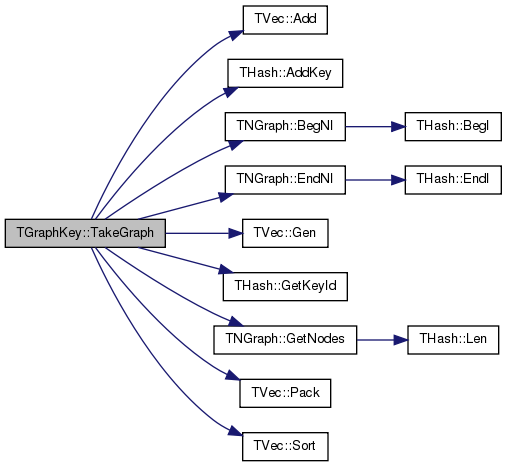Here is the caller graph for this function:void TGraphKey::TakeGraph ( const PNGraph & Graph, TIntPrV & NodeMap )

Creates a key from a given directed graph.

Parameter NodeMap stores the correspondence of old to new node Ids (0...N-1). Does not create a graph signature. Nodes are renumbered.

Definition at line 74 of file ghash.cpp.

74  {
75  TIntSet NodeIdH;
76  int n = 0;
77  NodeMap.Gen(Graph->GetNodes(), 0);
78  for (TNGraph::TNodeI NI = Graph->BegNI(); NI < Graph->EndNI(); NI++, n++) {
81  }
82  Nodes = Graph->GetNodes();
83  EdgeV.Gen(Nodes, 0);
84  for (TNGraph::TNodeI NI = Graph->BegNI(); NI < Graph->EndNI(); NI++) {
85  const int NewNId = NodeIdH.GetKeyId(NI.GetId());
86  for (int i = 0; i < NI.GetOutDeg(); i++) {
88  }
89  }
90  EdgeV.Sort(true);
91  EdgeV.Pack();
92 }
TPair< TInt, TInt > TIntPr
Definition: ds.h:83
int GetKeyId(const TKey &Key) const
Definition: shash.h:1328
TInt Nodes
Definition: ghash.h:12
void Sort(const bool &Asc=true)
Sorts the elements of the vector.
Definition: ds.h:1318
Definition: shash.h:1254
TIntPrV EdgeV
Definition: ghash.h:13
void Pack()
Reduces vector capacity (frees memory) to match its size.
Definition: ds.h:1057
Node iterator. Only forward iteration (operator++) is supported.
Definition: graph.h:383
void Gen(const TSizeTy &_Vals)
Constructs a vector (an array) of _Vals elements.
Definition: ds.h:523
Adds a new element at the end of the vector, after its current last element.
Definition: ds.h:602

Here is the call graph for this function: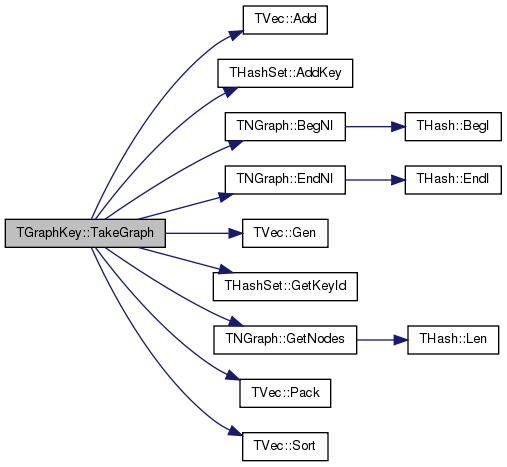void TGraphKey::TakeSig ( const PNGraph & Graph, const int & MnSvdGraph, const int & MxSvdGraph )

Creates a signature for a given directed graph.

The function only creates the signature vector but does not copy the graph.

Definition at line 94 of file ghash.cpp.

Referenced by TGHash< TDat >::AddKey(), TGHash< TDat >::GetNodeMap(), and TGHash< TDat >::IsGetKeyId().

94  {
95  const int Edges = Graph->GetEdges();
96  Nodes = Graph->GetNodes();
97  VariantId = 0;
98  SigV.Gen(2+Nodes, 0);
99  // degree sequence
100  TIntPrV DegV(Nodes, 0);
101  for (TNGraph::TNodeI NodeI = Graph->BegNI(); NodeI < Graph->EndNI(); NodeI++) {
103  }
104  DegV.Sort(false);
105  // compose the signature: nodes, edges, sorted in- and out- degree sequence
108  for (int i = 0; i < DegV.Len(); i++) {
111  }
112  // singular values signature
113  // it turns out that it is cheaper to do brute force isomorphism
114  // checking than to calculate SVD and then check isomorphism
115  if (Nodes >= MnSvdGraph && Nodes < MxSvdGraph) {
116  // perform full SVD
118  TFltV SngValV;
119  TFltVV LSingV, RSingV;
120  TIntH NodeIdH;
122  for (TNGraph::TNodeI NodeI = Graph->BegNI(); NodeI < Graph->EndNI(); NodeI++) {
124  }
125  for (TNGraph::TNodeI NodeI = Graph->BegNI(); NodeI < Graph->EndNI(); NodeI++) {
126  const int NodeId = NodeIdH.GetKeyId(NodeI.GetId()) + 1;
127  for (int e = 0; e < NodeI.GetOutDeg(); e++) {
128  const int DstNId = NodeIdH.GetKeyId(NodeI.GetOutNId(e)) + 1; // no self edges
129  if (NodeId != DstNId) AdjMtx.At(NodeId, DstNId) = 1;
130  }
131  }
132  try { // can fail to converge but results seem to be good
134  } catch(...) {
135  printf("\n***No SVD convergence: G(%d, %d): SngValV.Len():%d\n", Nodes(), Graph->GetEdges(), SngValV.Len());
136  }
137  // round singular values
138  SngValV.Sort(false);
139  for (int i = 0; i < SngValV.Len(); i++) {
141  }
142  }
143  //printf("SIG:\n"); for (int i = 0; i < SigV.Len(); i++) { printf("\t%f\n", SigV[i]); }
144  SigV.Pack();
145 }
TPair< TInt, TInt > TIntPr
Definition: ds.h:83
TFltV SigV
Definition: ghash.h:14
TSizeTy Len() const
Returns the number of elements in the vector.
Definition: ds.h:575
TInt Nodes
Definition: ghash.h:12
Definition: dt.h:1386
static void Svd(const TFltVV &InMtx, TFltVV &LSingV, TFltV &SingValV, TFltVV &RSingV)
Definition: xmath.cpp:1232
void Sort(const bool &Asc=true)
Sorts the elements of the vector.
Definition: ds.h:1318
static const int RoundTo
Definition: ghash.h:9
static double Round(const double &Val)
Definition: xmath.h:16
int GetKeyId(const TKey &Key) const
Definition: hash.h:466
Definition: hash.h:373
TInt VariantId
Definition: ghash.h:15
void Pack()
Reduces vector capacity (frees memory) to match its size.
Definition: ds.h:1057
Node iterator. Only forward iteration (operator++) is supported.
Definition: graph.h:383
void Gen(const TSizeTy &_Vals)
Constructs a vector (an array) of _Vals elements.
Definition: ds.h:523
Adds a new element at the end of the vector, after its current last element.
Definition: ds.h:602

Here is the call graph for this function: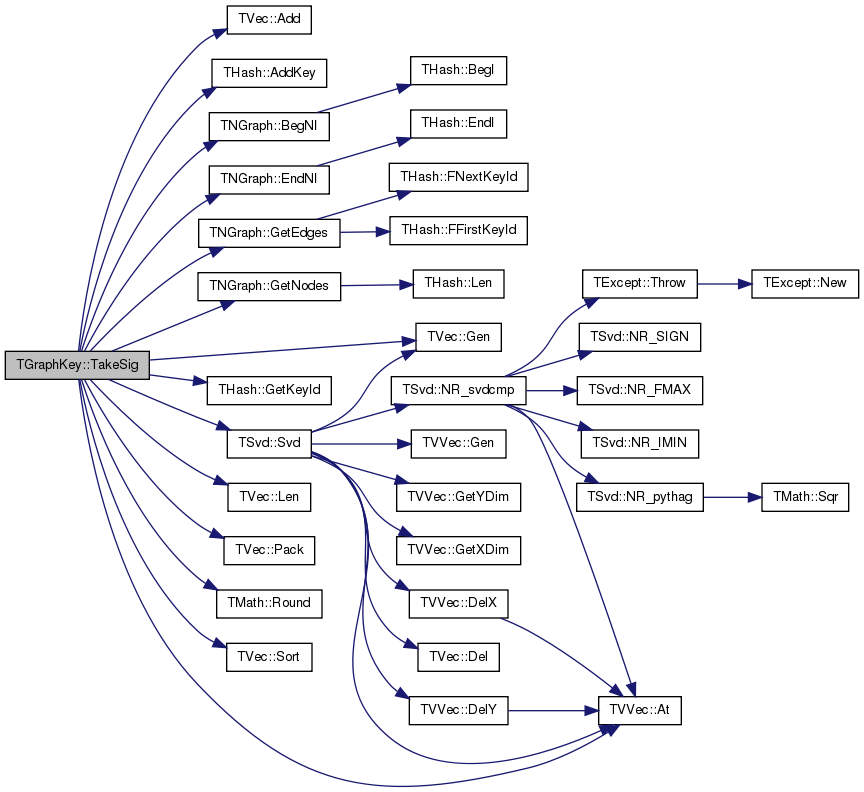Here is the caller graph for this function:## Member Data Documentation

 TIntPrV TGraphKey::EdgeV

Definition at line 13 of file ghash.h.

Referenced by GetNGraph(), IsIsomorph(), operator=(), Save(), SaveGViz(), SaveTxt(), and TakeGraph().

 TInt TGraphKey::Nodes

Definition at line 12 of file ghash.h.

Referenced by GetNodes(), IsIsomorph(), operator=(), Save(), SaveGViz(), TakeGraph(), and TakeSig().

 const int TGraphKey::RoundTo = 4
static

Definition at line 9 of file ghash.h.

Referenced by TakeSig(), and TGraphKey().

 TFltV TGraphKey::SigV

Definition at line 14 of file ghash.h.

Referenced by operator=(), operator==(), Save(), TakeSig(), and TGraphKey().

 TInt TGraphKey::VariantId

Definition at line 15 of file ghash.h.

Referenced by GetPrimHashCd(), GetVariant(), operator=(), operator==(), Save(), and TakeSig().

The documentation for this class was generated from the following files: